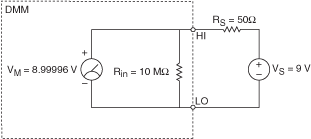# Input Resistance

NI Digital Multimeters Help (NI-DMM 18.1)

Edition Date: March 2018

Part Number: 370384V-01

»View Product InfoDownload Help (Windows Only)

Resistance is a measure of the opposition to the flow of current. The input resistance of the DMM can affect the accuracy of voltage measurements, particularly when the resistance of the source voltage is comparable to the input resistance of the DMM.

For example, assume a DMM with a 10 MΩ input resistance measures a 9 V source voltage with a series resistance of 50 Ω while in a 10 V DC range, as represented in the following figure.Using the following formula, you can determine the approximate value returned by the DMM:

VM = VS *[(Rin)/(RS + Rin)]

where VM is the voltage measured by the DMM, VS is source voltage, Rin is the input resistance of the DMM, and RS is external source resistance.

VM = (9 V * 10 M Ω)/(50 Ω + 10 M Ω)

VM = 8.99996 V

Another example is to assume a DMM with a 10 MΩ input resistance measures a 9 V source voltage with a series resistance of 1 M Ω while in a 10 V DC range, as represented in the following figure.Using the same formula, you can determine the approximate value returned by the DMM.

VM = (9 V * 10 M Ω)/(1 M Ω + 10 M Ω)

VM = 8.18182 V

When measuring the source voltage with a low source resistance, the DMM returns a value that was accurate to within ±0.0005%, or 5 ppm, and the same source voltage with a high resistance returns a value that was accurate to within ±10%, or 100,000 ppm.

NI 4065 and NI 4070/4071/4072 users can select the input resistance for DC voltage measurements.